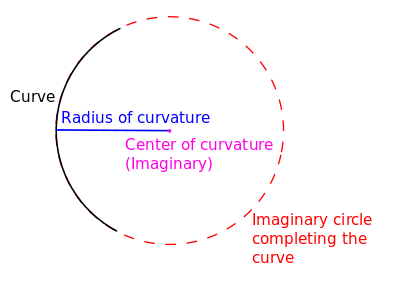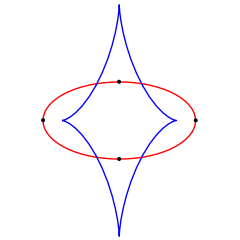Radius of curvature and center of curvature

In differential geometry, the radius of curvature, R, is the reciprocal of the curvature. For a curve, it equals the radius of the circular arc which best approximates the curve at that point. For surfaces, the radius of curvature is the radius of a circle that best fits a normal section or combinations thereof.

## Definition

In the case of a space curve, the radius of curvature is the length of the curvature vector.

In the case of a plane curve, then R is the absolute value ofwhere s is the arc length from a fixed point on the curve, φ is the tangential angle and κ is the curvature.

If the curve is given in Cartesian coordinates as y(x), then the radius of curvature is (assuming the curve is differentiable up to order 2):and |z| denotes the absolute value of z.

If the curve is given parametrically by functions x(t) and y(t), then the radius of curvature isHeuristically, this result can be interpreted as## Formula

If γ : ℝ → ℝn is a parametrized curve in n then the radius of curvature at each point of the curve, ρ : ℝ → ℝ, is given by.

As a special case, if f(t) is a function from to , then the curvature of its graph, γ(t) = (t, f(t)), is### Derivation

Let γ be as above, and fix t. We want to find the radius ρ of a parametrized circle which matches γ in its zeroth, first, and second derivatives at t. Clearly the radius will not depend on the position γ(t), only on the velocity γ′(t) and acceleration γ″(t). There are only three independent scalars that can be obtained from two vectors v and w, namely v · v, v · w, and w · w. Thus the radius of curvature must be a function of the three scalars |γ2(t)|, |γ2(t)| and γ′(t) · γ″(t).

The general equation for a parametrized circle in n iswhere c ∈ ℝn is the center of the circle (irrelevant since it disappears in the derivatives), a,b ∈ ℝn are perpendicular vectors of length ρ (that is, a · a = b · b = ρ2 and a · b = 0), and h : ℝ → ℝ is an arbitrary function which is twice differentiable at t.

The relevant derivatives of g work out to beIf we now equate these derivatives of g to the corresponding derivatives of γ at t we obtainThese three equations in three unknowns (ρ, h′(t) and h″(t)) can be solved for ρ, giving the formula for the radius of curvature:or, omitting the parameter t for readability,## Examples

### Semicircles and circles

For a semi-circle of radius a in the upper half-planeAn ellipse (red) and its evolute (blue). The dots are the vertices of the ellipse, at the points of greatest and least curvature.

For a semi-circle of radius a in the lower half-planeThe circle of radius a has a radius of curvature equal to a.

### Ellipses

In an ellipse with major axis 2a and minor axis 2b, the vertices on the major axis have the smallest radius of curvature of any points, R = b2/a; and the vertices on the minor axis have the largest radius of curvature of any points, R = a2/b.

## Applications

### Stress in semiconductor structures

Stress in the semiconductor structure involving evaporated thin films usually results from the thermal expansion (thermal stress) during the manufacturing process. Thermal stress occurs because film depositions are usually made above room temperature. Upon cooling from the deposition temperature to room temperature, the difference in the thermal expansion coefficients of the substrate and the film cause thermal stress.

Intrinsic stress results from the microstructure created in the film as atoms are deposited on the substrate. Tensile stress results from microvoids in the thin film, because of the attractive interaction of atoms across the voids.

The stress in thin film semiconductor structures results in the buckling of the wafers. The radius of the curvature of the stressed structure is related to stress tensor in the structure, and can be described by modified Stoney formula. The topography of the stressed structure including radii of curvature can be measured using optical scanner methods. The modern scanner tools have capability to measure full topography of the substrate and to measure both principal radii of curvature, while providing the accuracy of the order of 0.1% for radii of curvature of 90 meters and more.